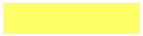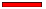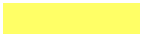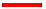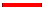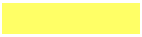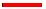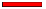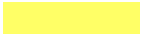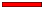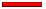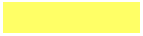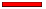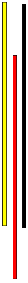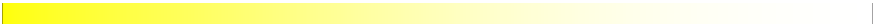EOS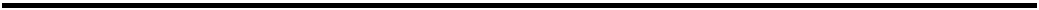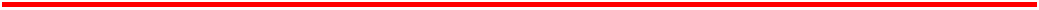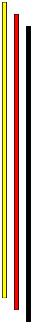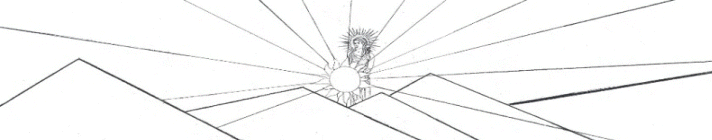Page 1 Momentum Derivation of the Rocket Equation Thrust   Page 2 Speed of the Rocket During the Burn   Page 3 Rocket Acceleration Position as a Function of Time   Page 4 Gravity   Page 5 Saturn V Data Stage 1 Calculation   Page 6 Saturn V Stage 2, Stage 3 Calculations   Page 7 Saturn V Photo Gallery   Page 8 Saturn V Photo Gallery   Page 9 Equation Review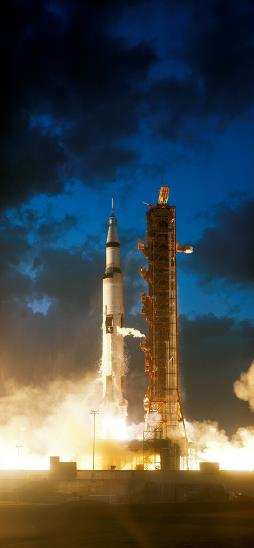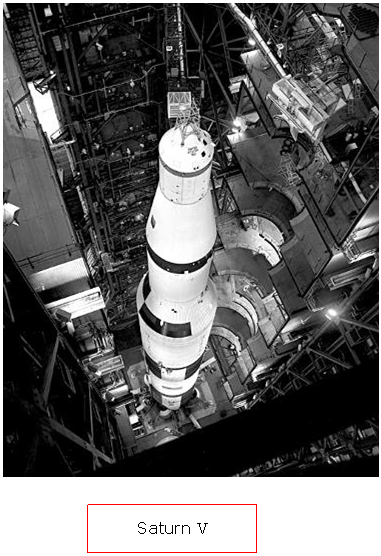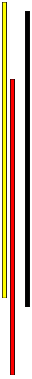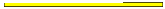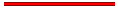A        Begin the analysis of rocket motion with momentum, p. For an object with particle-like motion, meaning without any rotation or internal vibrations, the momentum is just the object’s mass, m, times its velocity, v.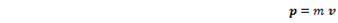Momentum can be a very useful concept because it may be conserved - under certain circumstances.  Velocity is a vector, so momentum is as well and it points in the same direction as velocity.  Mass is a scalar.  The momentum of a system of particles is the sum of the momentum of individual particles.   The purpose here is to write the rocket equation by finding the time-rate-of-change of momentum.  In order to do this, we use the operator d/dt  on p.  For the case such that mass is constant,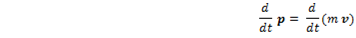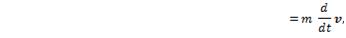but since acceleration is the time-rate-of-change of velocity,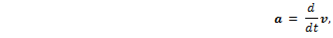we haveThe rocket and the expelled gas are considered together - as a system.  The total momentum of the system is the sum of the momentum of the exhaust gas and the momentum of the rocket - in opposite directions, of course.  Here, the term “rocket” refers to all of the mass except the exhaust gas: the rocket body (sometimes called “frame”), nose cone, astronauts, control systems, motor, payload, unspent fuel, and anything else carried along with the motion.   In one dimension we can drop the vector notation and write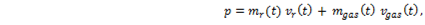where              mr(t) and vr(t) are the mass and velocity of the rocket at time t, and                       mgas(t) and vgas(t) are the mass and velocity of the expelled gas at time t.                       The quantities vr(t), mr(t), and mgas(t) vary with time as long as the fuel is burning; not only is the rocket speeding up, but the mass of the rocket is decreasing and the mass of the exhaust gas is increasing. Although vgas(t) was written for the general case as a function of time, in this case it isn’t, really.  Once the gas is expelled from the rocket motor it has a constant velocity because there is no longer a force acting on it.  Therefore, the explicit notation can be dropped and the gas velocity written as vgas.
 B        Thrust Momentum is conserved if there is no external force acting on the system; this means that there is no change in the total momentum.  Since the external force is zero,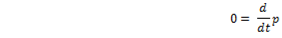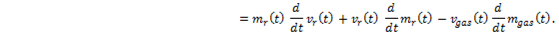Notice that as the fuel burns the mass lost by the rocket is the same amount of mass gained by the expelled gas, so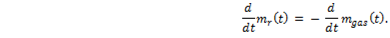Substitute this into the expression above to obtain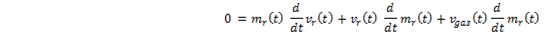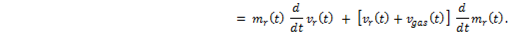Or,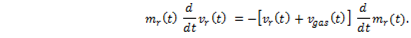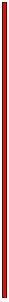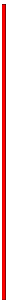webpages and Eos image copyright 2020  M Nealon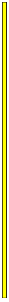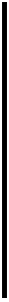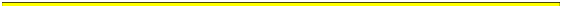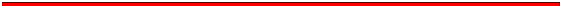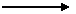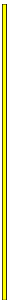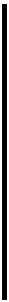That is Isaac Newton’s second law of motion.  The force on the object is the time-rate-of-change of its momentum: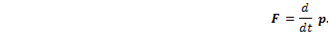For rockets, the fuel is – initially, anyway - a large proportion of the total mass.  As the fuel burns the total mass of the rocket decreases, so “the case such that the mass is constant” doesn’t hold, even approximately, for typical solid or liquid propellant rockets.  Nuclear powered rockets are another matter altogether.   As the nozzle of the rocket motor forces the high pressure gas in a particular direction, the gas exerts a force on the rocket in the opposite direction, accelerating the rocket as long as the fuel is being burned.   Generally, the term “motor” is used for solid propellant rockets and “engine” is used for liquid propellant rockets.  Once a solid fuel rocket motor is ignited it cannot be turned off — usually; the fuel burns all in one go.  Liquid fuel rocket engines may be switched off by a valve control mechanism.
 The two velocities vr (t) and vgas are measured relative to an outside observer, that is, someone not on the rocket. Now, here is a property of our favorite operator: acting on the product of two functions of time, say, g(t) and h(t), the operator gives the sum: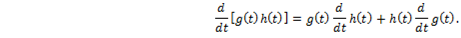Recalling that vgas is independent of time, applying this so-called product rule to the expression for momentum gives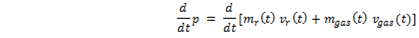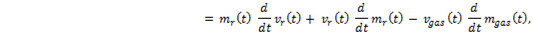where the negative sign is inserted into the equation in order to indicate that the gas velocity is in the opposite direction from the rocket velocity, and that vgas is taken as a positive quantity.  This is the celebrated rocket equation.   For the case at hand, we have no gravity, no atmospheric drag, and the rocket nozzle directs all of the exhaust gas in a single direction; i. e., no dispersion angle.
 Keep in mind that the velocities are relative to an outside observer in an inertial frame.  Velocities add, so that the velocity of the gas relative to the rocket, called the exhaust velocity, is vexhaust =  vr(t) + vgas.  The exhaust velocity is measured relative to the rocket.  Generally, rocket motors are designed so that the exhaust velocity is constant by making the pressure in the combustion chamber constant throughout the burn.   So we have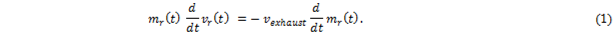The rate-of-change of mass of the rocket is negative; in other words, the mass of the rocket is decreasing, so let R be the rate of fuel burning, with R a positive quantity.  Then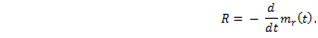So now,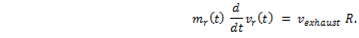Let vexhaust R = T. This is the force, known as thrust, acting on the rocket by the gas being expelled.  Newton’s second law may be written as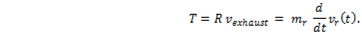The thrust is constant during the burn if R and vexhaust are constant, which is usually the case.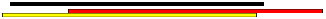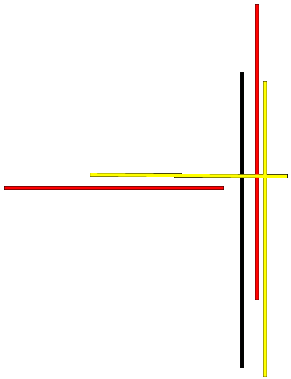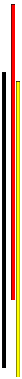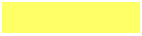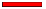1 Rocket Science